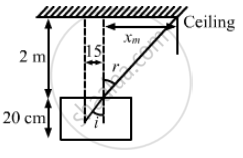Department of Pre-University Education, KarnatakaPUC Karnataka Science Class 12
Advertisement Remove all ads

# A Container Contains Water up to a Height of 20 Cm and There is a Point Source at the Centre of the Bottom of the Container. a Rubber Ring of Radius R Floats Centrally on the Water - Physics

Sum

A container contains water up to a height of 20 cm and there is a point source at the centre of the bottom of the container. A rubber ring of radius r floats centrally on the water. The ceiling of the room is 2.0 m above the water surface. (a) Find the radius of the shadow of the ring formed on the ceiling if r = 15 cm. (b) Find the maximum value of r for which the shadow of the ring is formed on the ceiling. Refractive index of water = 4/3.

Advertisement Remove all ads

#### Solution

Given,
Height (h) of the water in the container = 20 cm
Ceiling of the room is 2.0 m above the water surface.
Radius of the rubber ring = r
Refractive index of water = 4/3From the figure, we can infer:
$\sin i = \frac{15}{25}$
Using Snell's law, we get:
$\frac{\sin i}{\sin r} = \frac{1}{\mu} = \frac{3}{4}$
$\Rightarrow \sin i = \frac{4}{5}$
From the figure, we have:

$\tan r = \frac{x}{2}$
$So,$
$\sin r = \frac{\tan r}{\sqrt{1 + \tan^2 r}}$
$= \frac{\frac{x}{2}}{\sqrt{1 + \frac{x^2}{4}}}$
$\Rightarrow \frac{x}{\sqrt{4 + x^2}} = \frac{4}{5}$
$\Rightarrow 25 x^2 = 16(4 + x^2 )$
$\Rightarrow 9 x^2 = 64$
$\Rightarrow x = \frac{8}{3} m$

Total radius of the shadow = $\frac{8}{3} + 0 . 15 = 2 . 81 m$
(b)
Condition for the maximum value of r:
Angle of incidence should be equal to the critical angle, i.e., $i = \theta_c$
Let us take R as the maximum radius.
Now,

$\sin \theta_c = \frac{\sin \theta_c}{\sin r}$

$= \frac{R}{\sqrt{R^2 + 20}} = \frac{3}{4} (\sin r = 1)$

$\Rightarrow 16 R^2 = 9 R^2 + 9 \times 400$

$\Rightarrow 7 R^2 = 9 R^2 + 9 \times 400$

$\Rightarrow R = 22 . 67 cm$

Is there an error in this question or solution?
Advertisement Remove all ads

#### APPEARS IN

HC Verma Class 11, 12 Concepts of Physics 1
Chapter 18 Geometrical Optics
Q 33 | Page 414
Advertisement Remove all ads
Advertisement Remove all ads
Share
Notifications

View all notifications

Forgot password?